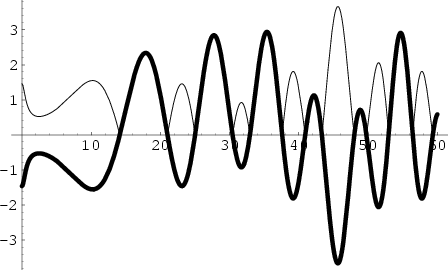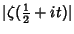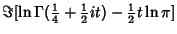## Riemann-Siegel FunctionsFor a Real Positive, the Riemann-Siegelfunction is defined byThe top plot superposes(thick line) on, whereis the Riemann Zeta Function.The Riemann-Siegel theta function appearing above is defined byThese functions are implemented in Mathematica(Wolfram Research, Champaign, IL) as RiemannSiegelZ[z] and RiemannSiegelTheta[z], illustrated above.